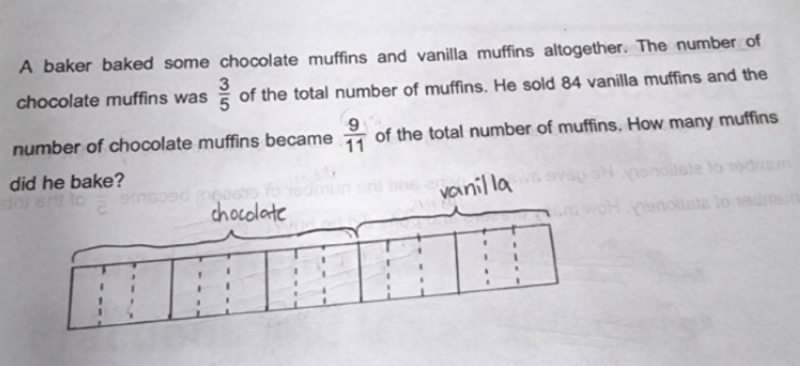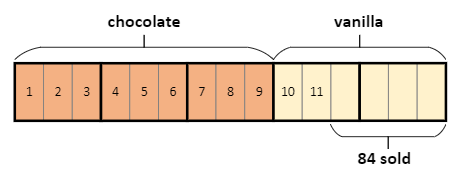# Question```Is that the child's usual standard of drawing block diagrams?
I must commend that it is very neat! Awesome!

Anyway the block diagram was very close to the solution.
Here is how I would continue:Reasoning:
- the chocolate section is made up of 9 bars, so we can
count 2 of the vanilla bars as 10 and 11
- that makes chocolate 9/11 of total remaining

So the 4 bars is the 84 sold muffins. Each bar is 84/4 = 21.
Total muffins baked is 15 bars = 15 x 21 = 315.

CHECK
chocolate           vanilla           TOTAL
Before     3/5 x 315 = 189     2/5 x 315 = 126   315
After      189                 126 - 84 = 42     231
Fraction = 189 / 231 = 9/11

```
0 Replies 2 Likes

Chocolate Muffins                Vanilla Muffins                  Total

At first                   3u                                             2u                                  5u

Sold                                                                       -84

In the end             9p                                            2p

We know that the chocolate muffins remain unchanged so the ratios are equal.

So  9p = 3u => p =⅓ u

2u – 84 = 2(⅓ u )           (multiply both sides by 3)

6u – 252 = 2u

u = 252/4 =  63

Hence the total muffins baked = 5u = 5 x 63 = 315

0 Replies 1 Like